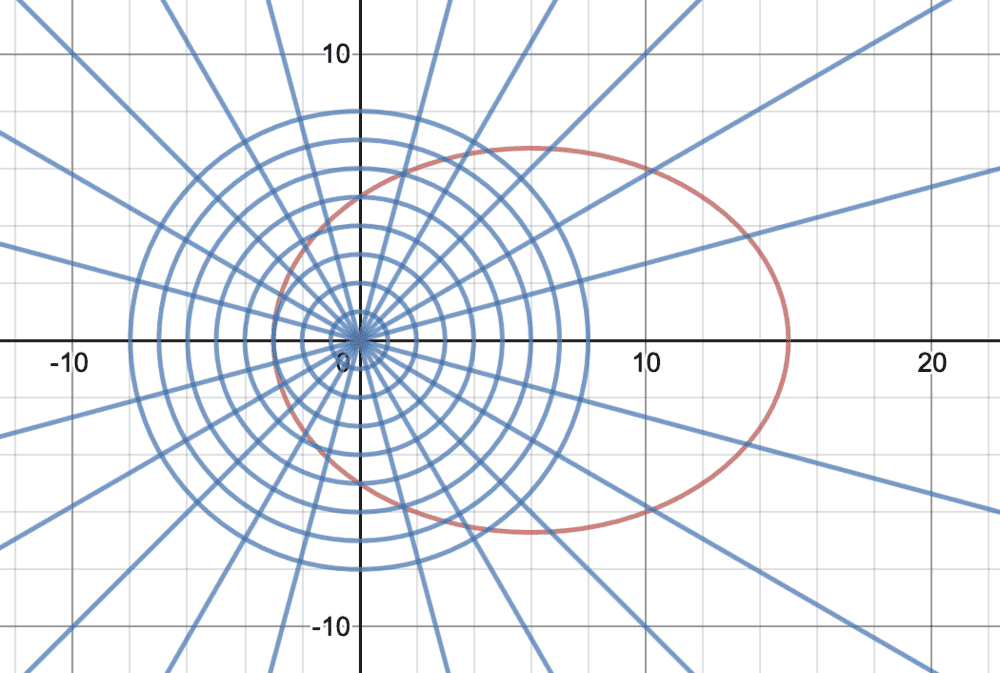# Polar equation of conics

Homework Statement:
Conic: r = 15/[3-2cos(theta)]
Relevant Equations:
conic general form when vertical directrix to left of pole:
r = ed/[1-e*cos(theta)], where d is the distance between focus at pole and the directrix
The text says that the following conic, r = 15/[3-2cos(theta)], can be rearranged to 5/[1-(2/3)cos(theta)]. The graph of the conic is an ellipse with e=2/3.
Then it says that the vertices lie at (15,0) and (3,pi). How did they find the vertices? Thanks.

docnet
Gold Member
hello I_love_science. Here is a graph of your polar equation for reference.If you notice the ellipse is symmetric about an axis, you can plug in certain values of ##θ## to determine the vertices in polar coordinates ##(r, θ)## and convert them to ##(x, y)## coordinates. In this example, the ellipse is symmetric about the x axis, which makes finding vertices more streamlined.

If the ellipse was not symmetric about the x or y axis, then my first step would be to find the axis of symmetry.